3.5 Types of Matrices:

Definitions:

 ‘Square Matrix’ is a matrix whose number of rows are same as number of columns. Square matrix’s order is represented by m (m x m) A=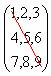order :3X3 B=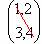order: 2X2 The Principal diagonal elements (from top left corner to bottom right corner) of matrix A are {1,5,9}     The Principal diagonal elements (from top left corner to bottom right corner) of matrix A are {1,4} A=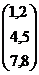order :3X2 B=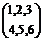order :2X3 Note Matrix A is  3X2   and Matrix B is 2X3   Since these two are not square matrices, we cannot identify their diagonal elements. A matrix whose principal diagonal elements are non zero and all other elements are zero is called ‘Diagonal matrix.’ A=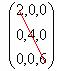Principal diagonal elements are {2,4,6} B=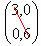Principal diagonal elements are {2,4,6} Observe that in both A and B except for diagonal elements all other elements are zero. A ‘Scalar matrix’  is a diagonal matrix whose principal diagonal elements are equal A=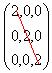Principal diagonal elements are {2,2,2}  B=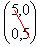Principal diagonal elements are {5,5} An ‘Identity matrix’  is a diagonal matrix whose principal diagonal elements are 1. A=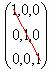Principal diagonal elements are {1,1,1} B=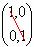Principal diagonal elements are {1,1} ‘Symmetric matrix’ is a square matrix whose elements are symmetric (same) with respect to principal diagonal elements. (Mirror copy with respect to principal diagonal). A=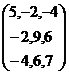Principal diagonal elements are {5, 9,6} B=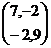Principal diagonal elements are {7, 9} Notice that in A the elements on both the sides of principal diagonal are same   {-2,-2},{-4,-4},{6,6}.     Notice that in B the elements on both the sides of principal diagonal are same   {-2,-2}. Skew symmetric matrix’ is a square matrix whose elements are symmetric with respect to the principal diagonal with opposite sign and principal diagonal elements are zero A=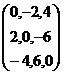Principal diagonal elements are {0,0,0}   B=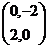Principal diagonal elements are {0,0} Notice that in A the elements on both the sides of principal diagonal are same with opposite sign {-2,2},{4,-4},{-6,6}.)   Notice that in B the elements on both the sides of principal diagonal are same with opposite sign {-2,2}.) A=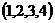order 1X4 B=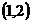order 1X2 Row matrix’ is a matrix which has only one row and is of the order (1 x n) A=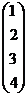order: 4X1 B=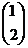order :  2X1 ‘Column  matrix’ is a matrix which has only one column  and is of order (m x 1) A=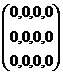order 3X4  B=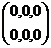order 2X3 ‘Zero  matrix’ is a matrix whose elements are all zeros.   It need not be a square matrix

 A=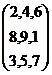B=Then A=B Two matrices are said to be ‘equal’ if and only if they are of same order and corresponding elements are equal. A=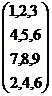B =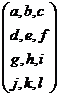If A=B, then a=1, b=2,c=3,d=4,e=5,f=6,g=7,h=8,i=9,j=2,k=4,l=6.  Rows  Columns ‘Transpose of a matrix’   is obtained by converting elements of rows in to columns and elements of columns in to rows. Transpose of A is denoted by A1. A=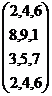order   4X3   A1=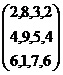order   3X4 Rows:  {2,4,6},{8,9,1},{3,5,7},{2,4,6}. Columns:  {2,8,3,2},{4,9,5,4},{6,1,7,6}       Rows:  {2,8,3,2},{4,9,5,4},{6,1,7,6}. Columns:  {2,4,6},{8,9,1},{3,5,7},{2,4,6}

3.5 Summary of learning

 No Points studied 1     2 Types of matrices - Square matrix, rectangular matrix, diagonal matrix, Symmetric matrix, Zero matrix, Skew matrix, Identity matrix Transpose of a matrix